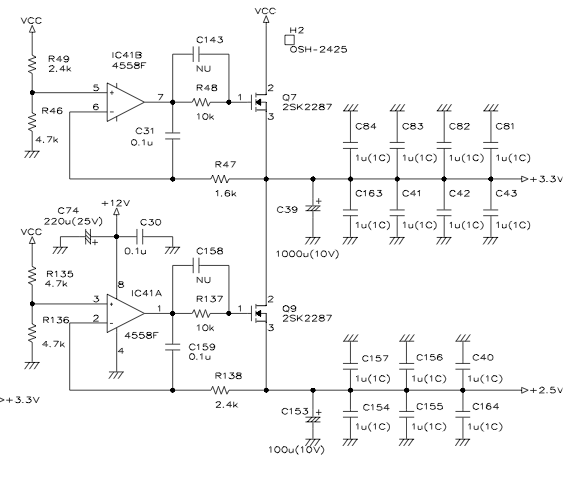# Is this an opamp voltage regulator?

I'm trying to understand the attached schematic, which I came across when repairing an oscilloscope.Am I right in thinking that this circuit is regulating VCC (5V) down to 3.3 and 2.5 volts? Why does the opamp use 12v rails rather than 5volts?

The non-inverting input to the opamp seems to be a voltage divider. I take this divider is used to provide a simple voltage reference?

Given that this is in an oscilloscope (a LeCroy LT342) is there any reason to use an opamp here rather than a voltage regulator?

One further thing that confuses me, is that on the board Q9 is not fitted, and has been replaced with a short between 2 of the pins. I can't understand how the circuit can work in this scenario, can anyone cast any light on this?

From my naive understanding, this seems like a bad design, and that it would be preferable to use a voltage regulator. What designed decisions might I have missed here?

• The lower V rails are linked ratiometrically to Vcc by the resistor ratios used in R49, R46 and R135, R136. If you increase or decrease the 5V rail by X% the other two rails will alter by the same percentage. This may be advantageous. Or not. Oct 15, 2014 at 12:30

They are regulators as you thought.

To properly control the MOSFET on each regulator, the gate needs to rise several volts above the source, probably over 6 volts greater than the source. If the source voltage is 3.3 volts the gate might need to rise as high as 9 or 10 volts when the MOSFET is driving a heavy load current. This means the op-amp MUST be powered from a supply greater than 10 volts.

The non-inverting input is the demand voltage for the regulator - for the 3V3 supply the non-inverting input will be 3.3 volts and ditto the 2V5 supply.

The MOSFET is rated at 20 amps and this means the supply current to the circuit might be in the realm of 5 to 8 amps - try and find a linear voltage regulator that would fit the bill an be a low drop-out type - realistically with a drain voltage of fractionally above 3.3 volts the output would still be in regulation. This is of course due to the op-amp being powered from 12 volts.

As to whether it is a bad design, I'd say not BUT I don't know the full spec of the circuit.

• I see, thanks! That really helps me understand the design considerations better! Oct 15, 2014 at 8:36
• Thanks again for you help. I wrote up my notes based on my new understanding of this circuit here: 41j.com/blog/2014/10/opamp-as-a-voltage-regulator One remaining question I had was the purpose of R138. It seems like it's a feedback resistor but I don't think that makes sense? Oct 15, 2014 at 12:47
• I would say R138 and C159 form a low-pass filter on the feedback path. I don't know the age of this scope but at a guess, this circuit was less expensive than using a low-dropout regulator capable of handling the required current at the time it was designed? Oct 15, 2014 at 13:13

Q9 is not fitted, and has been replaced with a short between 2 of the pins